Courses

# Major Organic Chemistry

## 60 Questions MCQ Test MOCK Test Series for IIT JAM Chemistry | Major Organic Chemistry

Description
This mock test of Major Organic Chemistry for Chemistry helps you for every Chemistry entrance exam. This contains 60 Multiple Choice Questions for Chemistry Major Organic Chemistry (mcq) to study with solutions a complete question bank. The solved questions answers in this Major Organic Chemistry quiz give you a good mix of easy questions and tough questions. Chemistry students definitely take this Major Organic Chemistry exercise for a better result in the exam. You can find other Major Organic Chemistry extra questions, long questions & short questions for Chemistry on EduRev as well by searching above.
QUESTION: 1

Solution:
QUESTION: 2

Solution:
QUESTION: 3

### The reactivity of compound I-IV with maleic anhydride (V) follows the order:  (I)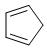(II)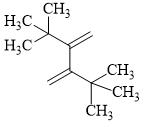(III)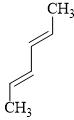(IV)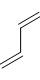(V)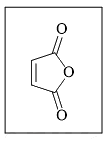Solution:
QUESTION: 4

Major product formed in the following reaction is: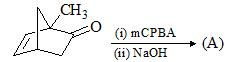Solution:
QUESTION: 5

The mechanism of the transformation involves: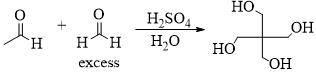Solution:
QUESTION: 6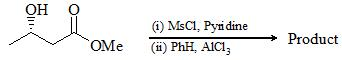Solution:
QUESTION: 7

The major product of the following reaction is: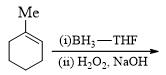Solution:
QUESTION: 8

Consider the following: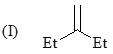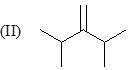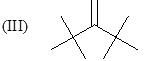Order of stretching frequency in IR-spectroscopy would be:

Solution:

Correct Answer :- a

Explanation : The stretching frequency increases with increase in the number of Pi bond which is present in the carbon bond. In case of triple bond there are two pi bond , in case of double bond there is only a single pi bond.

This is because with increase in the number of bond there is decrease in the atomic distance and hence there is more chance of stretching between the two atoms with respect to each other.

QUESTION: 9

The major product formed in the following reaction is: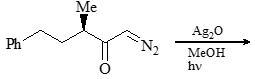Solution:
QUESTION: 10

The major product formed in the reaction given below is: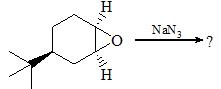Solution:
QUESTION: 11

Which is reducing sugar?

Solution:

Ans: c

Explanation: A reducing sugar is any sugar that is capable of acting as a reducing agent because it has a free aldehyde group or a free ketone group. Ketoses must first tautomerize to aldoses before they can act as reducing sugars. The common dietary monosaccharides galactose, glucose and fructose are all reducing sugars.

QUESTION: 12

The conditions A-B, required for the following pericyclic are: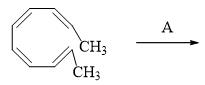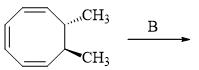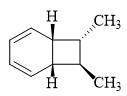Solution:
QUESTION: 13

In the following three step transformation, identify the correct combination product P, Q and R: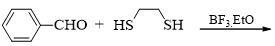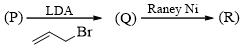Solution:
QUESTION: 14

Consider the given chemical reaction and predict B and C: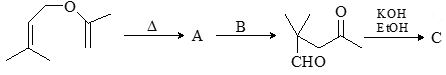Solution:
QUESTION: 15

Major product formed in the given reaction is: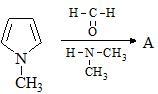Solution:
QUESTION: 16

Consider the following chemical reaction and choose the correct product: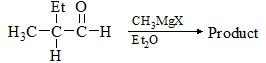Solution:
QUESTION: 17

Which of the following is diastereomer of the given compound?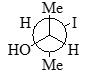Solution:
QUESTION: 18

Consider the following reactions for a compound with molecular formula C10H16: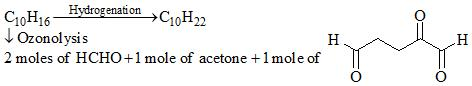C10H16 is a terpene called Myrcene and follows is isoprene rule. Hence, correct structure of Myrcene among three possible isomers of C10H16 is:

Solution:
QUESTION: 19

For the conversion of X to Y, the appropriate sequence of reaction is: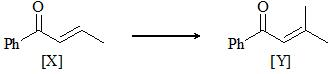Solution:
QUESTION: 20

Consider the following chemical reaction & predict the major product. [Major product has two geometrical isomers which are inter-convertible in presence of light]: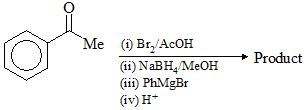Solution:
QUESTION: 21

The correct order of the basicity of following compounds is: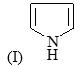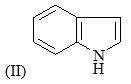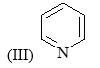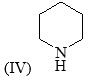Solution:
QUESTION: 22

Correct order of rate of hydrolysis for following compounds is: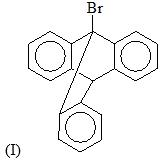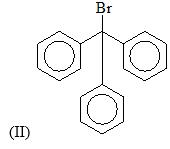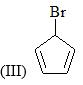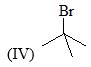Solution:
QUESTION: 23

Predict the product for the following sugar functionalization reaction: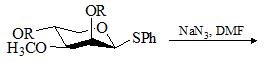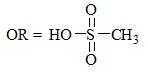Solution:
QUESTION: 24

Which of the following bases would give the high percentage of alk-1-ene from 2-bromo-2,3-dimethylbutane?

Solution:
QUESTION: 25

Choose the major product formed in given chemical reaction sequence: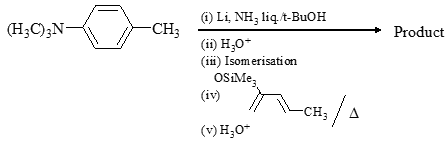Solution:
QUESTION: 26

What are the most likely products of the reaction shown below?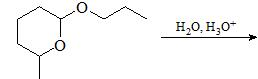Solution:
QUESTION: 27

Product [B] formed in the following reaction would be: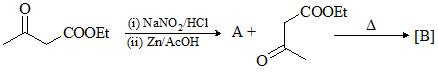Solution:
QUESTION: 28

Major product formed in the following chemical reaction would be?Solution:
QUESTION: 29

Consider the following chemical reaction and predict the product ‘D’: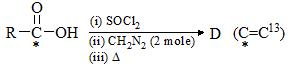Solution:
QUESTION: 30

Consider the following chemical reaction sequence. Predict the product ‘Z’: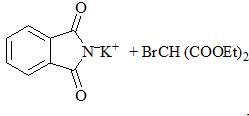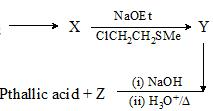Solution:
*Multiple options can be correct
QUESTION: 31

Which of the following is true?

Solution:
*Multiple options can be correct
QUESTION: 32

Choose the correct product(s):

Solution:
*Multiple options can be correct
QUESTION: 33

Which of the following compound do/es not show/s mutarotation?

Solution:
*Multiple options can be correct
QUESTION: 34

Consider the following chemical reaction and choose the correct products involved: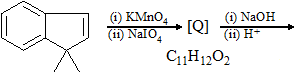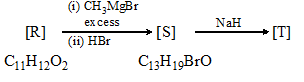Solution:
*Multiple options can be correct
QUESTION: 35

Product formed by P & Q can be differentiated by: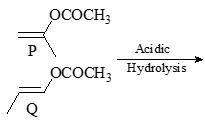Product formed by P & Q can be differentiated by:

Solution:
*Multiple options can be correct
QUESTION: 36

Optically pure ester (L) is hydrolysed in aq. acetic acid to form a recemical mixture of [M]. Choose correct statement(s):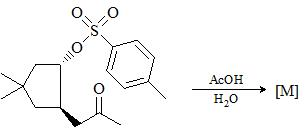Solution:
*Multiple options can be correct
QUESTION: 37

Which of the following reactions will form carbene:

Solution:
*Multiple options can be correct
QUESTION: 38

Consider the following chemical reaction and select the correct alternative(s):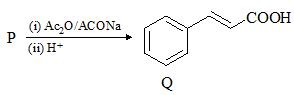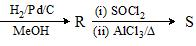Solution:

Option A and C is correct. D is wrong as on the reduction of cinnamic acid. Aldehyde will not be formed, only double bond will get reduced.

*Multiple options can be correct
QUESTION: 39

Which of the following compounds is achiral:

Solution:
*Multiple options can be correct
QUESTION: 40

Consider the reaction sequence: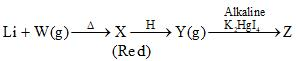Choose the correct option(s): (‘X’ is hydrolyzed to give ‘Y’)

Solution:
*Answer can only contain numeric values
QUESTION: 41

Compound 'X' containing chlorine on treatment with strong ammonia gives a solid 'Y' which is free from chlorine. 'Y' was analyzed and found to contain 44.31% C, 9.59% H and 19.50% N. ‘X’ reacts with Br2 and caustic soda to give a basic compound 'Z'. 'Z' give ethanol on treatment with HNO2. Find the total number of carbon atom(s) present in the empirical formula of 'Y':

Solution:
*Answer can only contain numeric values
QUESTION: 42

How many of the following compound(s) give(s) positive Haloform test?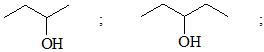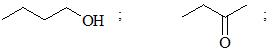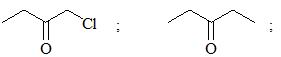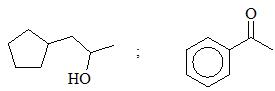Solution:
*Answer can only contain numeric values
QUESTION: 43

How many mole(s) of RMgX can be consumed in the reaction of following compound?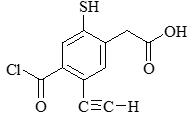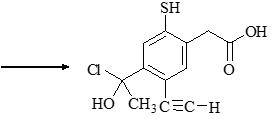Solution:
*Answer can only contain numeric values
QUESTION: 44

Calculate number of peptide(s) formed after treatment of following polypeptide with Chemotrypsin.:
Lys-Phe-Gly-Asp-Trp-Ser-Arg-Trp-Leu-His

Solution:
*Answer can only contain numeric values
QUESTION: 45

Find the degree of unsaturation in major product: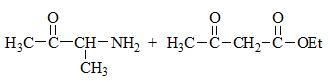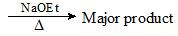Solution:
*Answer can only contain numeric values
QUESTION: 46

How many five membered ring(s) will be form in the following chemical reaction?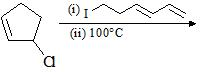Solution:
*Answer can only contain numeric values
QUESTION: 47

The number of resonance structures for product ‘N’ is: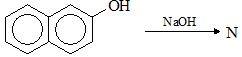Solution:
*Answer can only contain numeric values
QUESTION: 48

Number of compound(s) that will undergo Hoffman Bromamide reaction?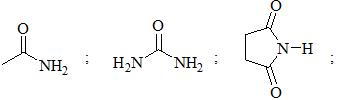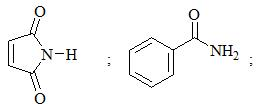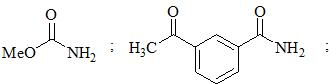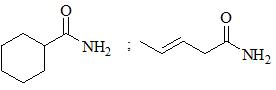Solution:
*Answer can only contain numeric values
QUESTION: 49

When methylglucopyranoside reacts with periodic acid, how many mole(s) of the oxidizing agent will be consumed per mole of the sugar?

Solution:
*Answer can only contain numeric values
QUESTION: 50

How many of the following heterocyclic compound(s)is/are aromatic?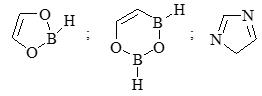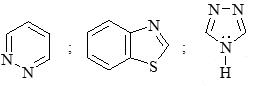Solution:

Except 3rd one all are aromatic heterocyclic compounds.

*Answer can only contain numeric values
QUESTION: 51

Number of correct chemical conversion among following is/are: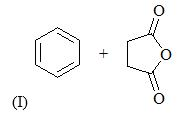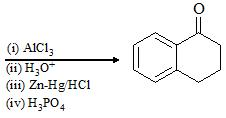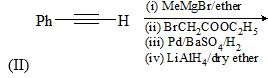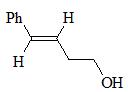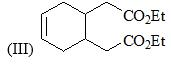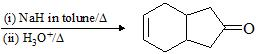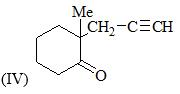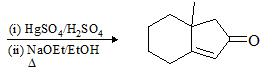Solution:
*Answer can only contain numeric values
QUESTION: 52

A chemist finds that the addition of (+)-epinepherine to the catalytic reduction of butan-2-on gives a product that is slightly optically active, with a specific rotation of +0.45°. Calculate the percentage of (+)-butan-2-ol formed in this reaction. If the specific rotation of enantiomerically pure (+)-butan-2-ol is + 13.5°: [Calculate up to 2 decimal place]

Solution:
*Answer can only contain numeric values
QUESTION: 53

Double bond which is most reactive for hydrogenation reaction with Wilkinson’s catalyst?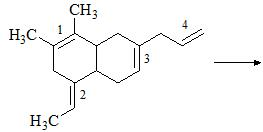Solution:
*Answer can only contain numeric values
QUESTION: 54

Calculate the sum of π bonds, Oxygen atom(s) and Nitrogen atom(s) in the final product of following chemical conversion: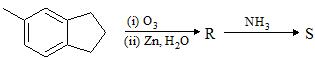Solution:
*Answer can only contain numeric values
QUESTION: 55

The number of chemical reaction(s) which represent correct product formation: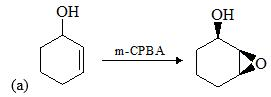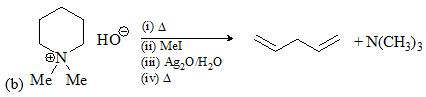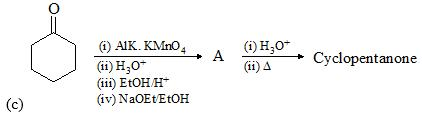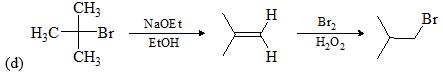Solution:
*Answer can only contain numeric values
QUESTION: 56

Consider the given chemical reaction and find the value of ‘X’: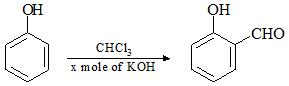Solution:
*Answer can only contain numeric values
QUESTION: 57

The alkyl halide C4H9Br(A) reacts with alcoholic KOH and gives an alkene (B), which reacts with bromide to give dibromide (C). (C) is transformed with KOH & sodamide to gas (D) which forms a precipitate when passed through an ammonical silver nitrate solution. Total number of sp3 carbon present in C and D would be:

Solution:
*Answer can only contain numeric values
QUESTION: 58

Number of sp2 hybridized carbon in the product would be?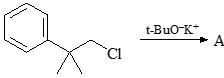Solution:
*Answer can only contain numeric values
QUESTION: 59

How many molecules of CO2 will be released in the following chemical reaction?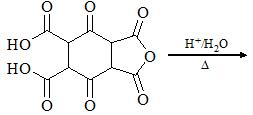Solution:
*Answer can only contain numeric values
QUESTION: 60

Sum of molar mass of the gaseous product(s) formed: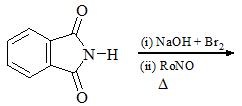Solution: